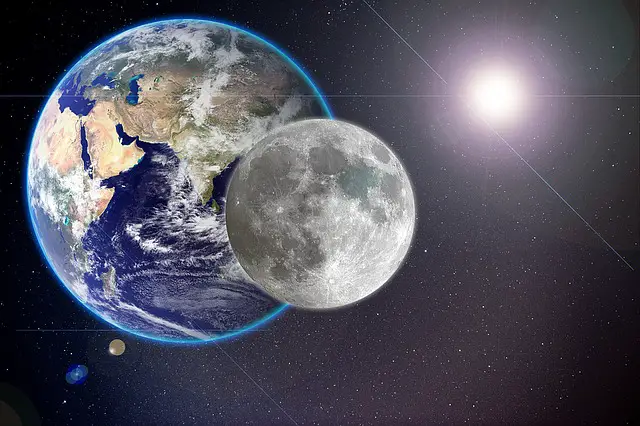# Kepler’s Third Law – statement, equation & derivation [class 11]

Last updated on June 4th, 2023 at 02:25 pm

We will derive the equation for Kepler’s Third Law using the concept of the Period of Revolution and the equation of orbital velocity. In this process, the equation of the Time Period Of Revolution of the Earth satellite would be derived as well. We’ll also solve sample numerical problems here using this law. This post is useful for class 11 students studying physics. So let’s start!

Kepler’s Third Law [statement]

Kepler’s Third Law or 3rd Law of Kepler is an important Law of Physics, which talks about the period of its revolution and how the period of revolution of a satellite depends on the radius of its orbit.

This law states that the square of the Orbital Period of Revolution is directly proportional to the cube of the radius of the orbit.

Time Period of Revolution of Earth’s satellite & derivation of its Equation

Say, the Period of revolution is T and the radius of the orbit is r.

And r = R + h = Radius of the earth + height of the satellite from the surface of the earth.

So the period of revolution = T = (2 π r) /V, where V is the Orbital Velocity of the satellite. …………. (1)

Now from the previous post on the Orbital Velocity of a Satellite, we know that orbital velocity can be expressed as V = R √(g/r) = R (g/r)1/2

Here g is the acceleration due to gravity and R is the radius of the earth …………….(2)

From equations 1 and 2, we get,

T = (2 π r)/V = [(2 π r)] /[R (g/r)(1/2)]
=[ 2π / R]. √[r3/g]

Here we get an important equation for the Period of Revolution of Earth satellite:

T = [ 2π / R]. √[r3/g] …………………(3) where R is the radius of the earth and r = R + h

In the next section, we will use the above equation to derive Kepler’s Third Law

Derivation of Kepler’s Third Law

The period of Revolution of Earth’s satellite can be expressed as
T = [ 2π / R]. √[r3/g] [derived above, see equation 3]

Now if we square both sides of the above equation we get the following:

T^2 =[ (4 . π2)/(R2)]. r3/g ……………………………(4)

Here, (4. π2)/(R2) and g are constant as the values of π (Pi), g, and R are not changing with time.

So we can say, T2 ∝ r3. …………….. (5) .[Kepler’s Third Law equation]

Here, Kepler’s Third Law equation says that the square of the Orbital Period of Revolution is directly proportional to the cube of the radius of the orbit.

If the orbit is not circular in the truest sense and rather elliptical, then this law states like this,
the square of the Orbital Period of Revolution varies with the cube of the semi-major axis of the orbit. This is known as Kepler’s Third Law.

From this equation of Kepler’s Third Law, it comes out clear that the mass of the object in the revolution has no effect on the Period of Revolution.

And this law is applicable to the revolution of any planet and satellite.

Now let’s solve a numerical problem using this formula, in the next paragraph.

Numerical Problem based on Kepler’s 3rd law

Two satellites Y and Z are rotating around a planet in a circular orbit. The radii of the orbits for Y and Z are 4R and R respectively. Now if the speed of Y satellite is 3v then what is the speed of satellite Z?

Solution:

We would use Kepler’s third law here.

Say, the radius of the orbit of Y and Z are Ry and Rz respectively.
As given data, Ry = 4R and Rz = R

From the 3rd Law of Kepler,
Ty^2/Tz^2 =Ry^3/Rz^3 = (4R)^3/R^3 = 64.  So, Ty/Tz = 8 ……………. (1)

Continuing with this equation (equation 1) and bringing in the orbital velocity in it we get:

(2 π Ry/Vy) / (2 π Rz/Vz) = 8

=> (Ry Vz) /(Rz Vy) = 8

=> (Ry/Rz).(Vz/Vy) = 8

=> (4R/R) .(Vz/Vy) = 8

=> (Vz/Vy) = 8/4 = 2

=> Vz = 2. Vy = 2. 3v = 6v

So the speed of satellite Z is 6v (answer)

Numerical Problem based on the Period of Revolution of the satellite

Q1) What is the value of the Period of Revolution of the Earth satellite? ignore the height of the satellite above the surface of the earth.
Given: gravitational acceleration (g) = 10 m/s^2. Radius of earth = R = 6400 km. Take π as 3.14

Solution:

Period of revolution = T = [ 2π / R]. √[r ^3/g]
Here r = R + h. As h is to be ignored according to the question, so r =R here.

So T = 2 π √(R/g) ……………. (1)

Given: R= 6400 km = 6400 X 10^3 meter. g = 10 m/s^2
Putting the values given, we get,

T = 2 X 3.14 X √ [(6400X10^3)/10] = 2 X 3.14 X 800 = 5024 seconds = 83.73 minutes.

Related Post:

escape velocity

Scroll to top
error: physicsTeacher.in